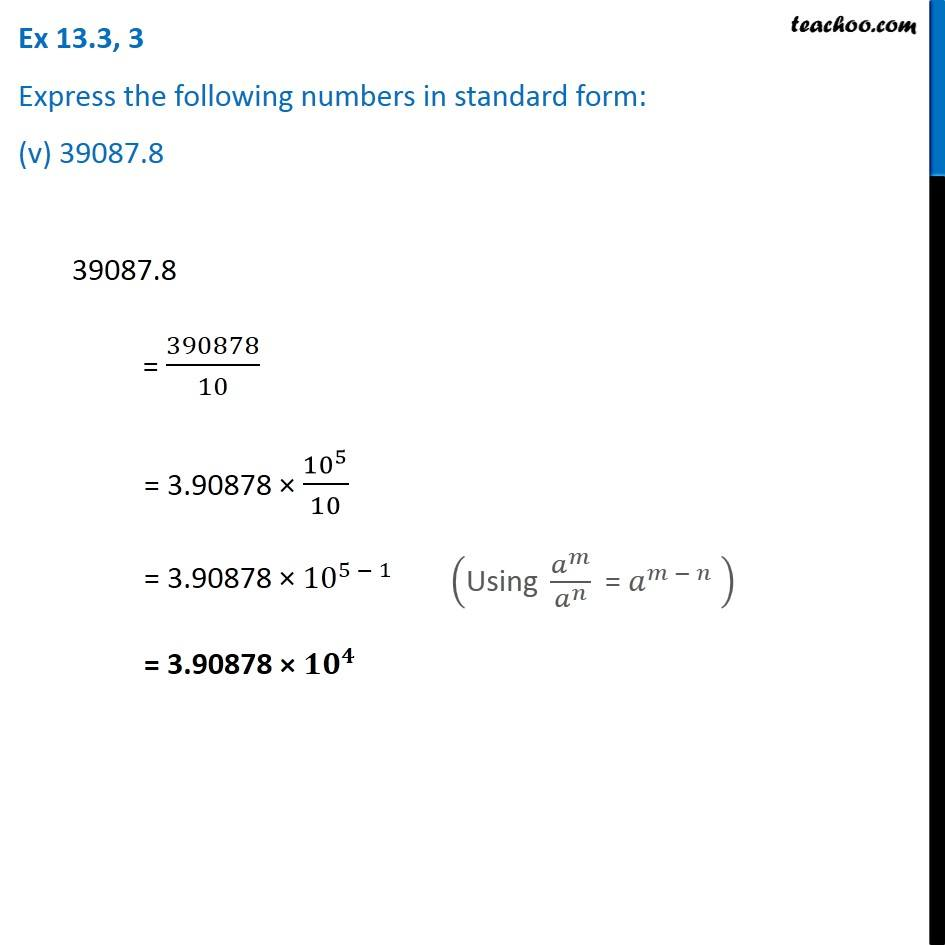Ex 13.3

Chapter 13 Class 7 Exponents and Powers
Serial order wiseGet live Maths 1-on-1 Classs - Class 6 to 12

### Transcript

Ex 13.3, 3 Express the following numbers in standard form: (v) 39087.8 39087.8 = 390878/10 = 3.90878 × 〖10〗^5/10 = 3.90878 × 〖10〗^(5 − 1) = 3.90878 × 〖𝟏𝟎〗^𝟒 ("Using " 𝑎^𝑚/𝑎^𝑛 " = " 𝑎^(𝑚 − 𝑛) " " )